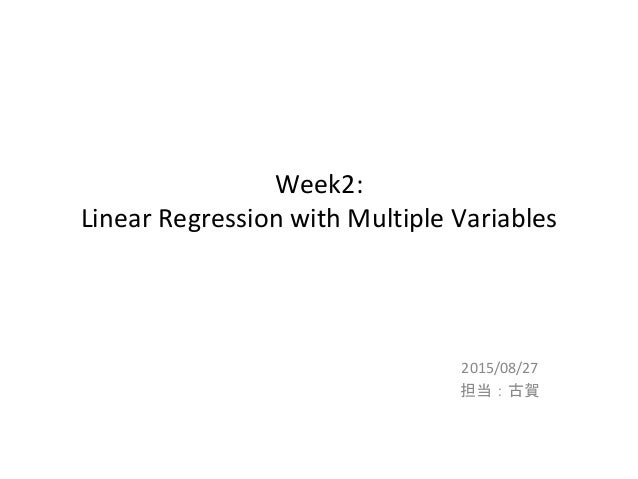Successfully reported this slideshow.Upcoming SlideShare
×

# Coursera Machine Learning Week2まとめ

3,772 views

Published on

Linear Regression with Multiple Variablesのまとめスライドです。

Coursera Machine Learning:
https://www.coursera.org/learn/machine-learning

・多変量線形回帰
・多変量線形回帰の最急降下法によるパラメータ推定
・特徴の正規化
・学習率の選定方法
・特徴の選定方法と多項式による回帰
・正規方程式によるパラメータ推定

Published in: Science
• Full Name
Comment goes here.

Are you sure you want to Yes No• Be the first to comment

### Coursera Machine Learning Week2まとめ

1. 1. Week2: Linear Regression with Multiple Variables 2015/08/27 担当：古賀
2. 2. Ｗｅｅｋ２で学習すること • 多変量線形回帰 • 多変量線形回帰の最急降下法によるパラメータ推定 • 特徴の正規化 • 学習率の選定方法 • 特徴の選定方法と多項式による回帰 • 正規方程式によるパラメータ推定 サマリー ＋ わかりにくかったところ を中心に解説します
3. 3. 単変量 vs. 多変量の線形回帰 仮説： 目的 関数： パラメータ： 最急降下法によるパラメータ推定法： 多変量単変量 又は、
4. 4. 多変量の線形回帰: J(Θ)
5. 5. 4 x 5 行列 要素数5のベクトル 要素数4のベクトル 要素数4のベクトル ベクトルの転置 × ベクトル ＝ スカラ スカラ 多変量の線形回帰: J(Θ)
6. 6. 多変量の線形回帰: min J(Θ) theta = theta – alpha * 1.0/m * X’ * (X*theta - y); (m x (n+1))の転置 m ベクトルn+1ベクトル
7. 7. 特徴の正規化 スケールが違いすぎる すべての特徴𝑥𝑖をおおよそ にしたい ・平均、標準偏差を使った正規化 例） 少し範囲を出ても良い。 𝑥𝑗 𝑖 ← 𝑥𝑗 𝑖 − 𝜇 𝑗 𝜎𝑗 𝑋1 以外の各要素に対して正規化する
8. 8. 学習率αの選定方法 ・αが小さすぎる： 収束に時間がかかる ・αが大きすぎる： 各繰り返しでJ(Θ)が減少し ない → J(Θ)が収束しない αの決め方 …, 0.001, 0.003, 0.01, 0.03, 0.1, 0.3, 1, ….. × 3 × 1/3
9. 9. 多項式回帰 多変量線形回帰のモデルを使って、多項式の回帰モデルを 作ることができる
10. 10. 正規方程式 Octave実装: pinv(X ' * X) * X' * y 最急降下法 ・学習率αを選ばなければならない ・繰り返し処理が必要 ・特徴数が多くても機能する
11. 11. %% Load Data data = load('ex1data2.txt'); X = data(:, 1:2); y = data(:, 3); 1. Xを正規化 2. 最急降下法でコスト関数最小化→パラメータ推定 （J(Θ)の値ををグラフで確認しつつ） 2’. 正規方程式による方法でパラメータ推定 11 Assignment(Multiple Variablesのみ) データの準備 ex1data2.txt 解く順序 ： 家のサイズ(feet^2) ベットルーム数 家の価格 問題：学習データを使って、家のサイズとベッドルーム数 から、家の価格を予測する
12. 12. 12 function [X_norm, mu, sigma] = featureNormalize(X) mu = mean(X); % (1 x 2) sigma = std(X); % (1 x 2) mu_m = ones(length(X), 1) * mu; % (m x 1)*(1 x 2)=(m x 2) sig_m = ones(length(X), 1) * sigma; % (m x 1)*(1 x 2)=(m x 2) X_norm = (X - mu_m) ./ sig_m; % OK (m x 2) %X_norm = (X - mu_m) / sig_m; % NG (m x m) end Feature Normalization featureNormalize.m [X mu sigma] = featureNormalize(X); X = data(:, 1:2); % (m x 2) y = data(:, 3); % (m x 1)
13. 13. 13 正規化前 正規化後
14. 14. 14 % Choose some alpha value alpha = 0.01; num_iters = 400; % Init Theta and Run Gradient Descent theta = zeros(3, 1); [theta, J_history] = gradientDescentMulti(X, y, theta, alpha, num_iters); Gradient Descent % Add intercept term to X X = [ones(m, 1) X]; % (m x 3) % Estimate the price of a 1650 sq-ft, 3 br house t = [1650, 3]; price = [1, (t-mu)./sigma] * theta; 正規化が必要（１以外） [X mu sigma] = featureNormalize(X); Xを正規化
15. 15. 15 function [theta, J_history] = gradientDescentMulti(X, y, theta, alpha, num_iters) % Initialize some useful values m = length(y); % number of training examples J_history = zeros(num_iters, 1); for iter = 1:num_iters h = X * theta; theta = theta - alpha * 1.0/m * X' * (h-y); % Save the cost J in every iteration J_history(iter) = computeCostMulti(X, y, theta); end end gradientDescentMulti.m
16. 16. 16 computeCostMulti.m function J = computeCostMulti(X, y, theta) % number of training examples m = length(y); % non-vectorized form %h = X*theta; %J = 1.0/(2.0*m) * sum((h - y).^2); % vectorized form J = 1.0/(2.0*m) * (X*theta-y)' * (X*theta-y); end
17. 17. 17 α=0.01
18. 18. α=0.01 α=0.03 α=0.1 α=1.4α=1.0 Selecting learning rates NG!
19. 19. 19 Normal Equations function [theta] = normalEqn(X, y) theta = pinv(X'*X) * X' * y; end % Calculate the parameters from the normal equation theta = normalEqn(X, y); % Estimate the price of a 1650 sq-ft, 3 br house price = [1, 1650, 3] * theta; normalEqn.m
20. 20. 20 最急降下法で パラメータ推定 正規方程式でパ ラメータ推定 結果